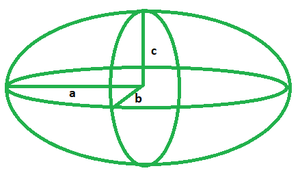Open in App
Not now

# Volume of an Ellipsoid Formula

• Last Updated : 31 Mar, 2022

An ellipsoid can be called a 3D equivalent of an ellipse. It can be derived from a sphere by contorting it using directional scaling or, more broadly, an interpolation conversion. An ellipsoid is evenly spaced at three coordinate axes that intersect at the centre.The above picture shows an ellipsoid, its three semi-axes denoted a, b and c are also shown. Ellipsoids occur in nature in the shape of watermelons, as well as the female reproductive organs and male urinary bladder. The study of the volume of the ellipsoid is necessary as it helps the doctors to calculate the volume of ovaries and urinary bladders.

Volume of Ellipsoid Formulawhere a, b and c are the semi- axes of the given ellipsoid.

### Sample Problems

Problem 1. Find the volume of an ellipsoid whose radii are 5, 6 and 11 cm.

Solution:

Given: a = 5 cm, b = 6 cm, c = 11 cm

Since,= 4 × 3.14 × 5 × 6 × 11/3

V = 1382.6 cm3

Problem 2. Find the volume of an ellipsoid whose radii are 3, 4 and 7 cm.

Solution:

Given: a = 3 cm, b = 4 cm, c = 7 cm

Since, V == 4 × 3.14 × 3 × 4 × 7/3

V = 351.68 cm3

Problem 3. Find the volume of an ellipsoid whose radii are 4, 7 and 11 cm.

Solution:

Given: a = 4 cm, b = 7 cm, c = 11 cm

Since, V == 4 × 3.14 × 4 × 7 × 11/3

V = 1289.49 cm3

Problem 4. Find the volume of an ellipsoid whose radii are 8, 6 and 14 cm.

Solution:

Given: a = 8 cm, b = 6 cm, c = 14 cm

Since, V == 4 × 3.14 × 8 × 6 × 14/3

V = 2813.44 cm3

Problem 5. Find the volume of an ellipsoid whose radii are 2, 6 and 8 cm.

Solution:

Given: a = 2 cm, b = 6 cm, c = 8 cm

Since, V == 4 × 3.14 × 2 × 6 × 8/3

V = 401.92 cm3

My Personal Notes arrow_drop_up